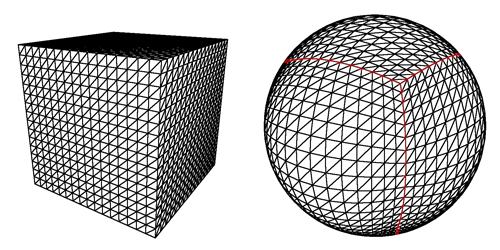# Generating Heightmaps for a Spherical Terrain

So what I’m trying to do is generate height maps for a spherical terrain and am having quite some trouble. My spherical terrain setup is pretty much a quadrilateralized spherical cube - a cube morphed into sphere (see first image below) that is subdivided into smaller patches by a quadtree (see second image below). To generate the heights for the height map I will be using a 3D perlin noise script. However, I don’t understand what positions I plug into the perlin noise to get the heights for the height map (each terrain patch will have its own height map) and afterwards apply the height map to the mesh to displace the vertices.My current code for generating the height map is as follows:

``````		Texture2D heightmap = new Texture2D(16, 16);

for(int x = 0; x < 16; x++){

for(int y = 0; y < 16; y++){

float theta = 2 * Mathf.PI * x;
float phi = Mathf.PI * y;

float _x = (Mathf.Cos(theta) * Mathf.Sin(phi) * radius);
float _y = (Mathf.Sin(theta) * Mathf.Sin(phi) * radius);
float _z = (-Mathf.Cos(phi)) * radius;

Vector3 pos = new Vector3(_x, _y, _z);
float noiseValue = (float)noise.GetValue(pos.x, pos.y, pos.z);

heightmap.SetPixel(x, y, Color.Lerp(Color.white, Color.black, (noiseValue+1)/2));

}

}
``````

This doesn’t work properly at all, I only showed it to further explain what I can’t figure out. The code above should be ignored for now. My end goal is to generate a height map with a size of 192x192 but I have to make some optimizations before I can do that. The vertex “resolution” for each terrain patch is 16x16 (289 vertices per patch), I don’t know if this helps or not though. To reiterate, my problem is that I don’t know how to generate a height map (texture) using a 3D perlin noise function so that the height map could be then mapped to the sphere to displace vertices.

EDIT: Couldn’t fit my response into a comment so I added it here…

There’s two methods for turning a cube into a sphere, one of them is simply:

``````Vector3 sphereVertex = cubeVertex.normalized * sphereRadius;
``````

which is relatively computationally inexpensive but it results in a planet mesh in which vertices near the “edges” of the spherical cube have higher density than the vertices near the centers of each face. Refer to the image above for an example. The second method requires a unit sized cube but generates a spherical cube with more equal vertex density:

``````float x = cubeVertex.x/cubeLength;
float y = cubeVertex.y/cubeLength;
float z = cubeVertex.z/cubeLength;
sphereVertex.x = x * Mathf.Sqrt(1 - ((y * y)/2) - ((z * z)/2) + (((y * y) * (z * z))/3));
sphereVertex.y = y * Mathf.Sqrt(1 - ((z * z)/2) - ((x * x)/2) + (((z * z) * (x * x))/3));
sphereVertex.z = z * Mathf.Sqrt(1 - ((x * x)/2) - ((y * y)/2) + (((x * x) * (y * y))/3));
``````

The three components of the cubeVertex are divided by the cubeLength in order to get the unit cube vertices, so the positions that are processed are already in unit space. When generating the evaluation positions or the “cubeVertex” positions you want to always use positions from the same cube otherwise you’ll end up with strange errors.

In my system each face of the spherical cube is technically a separate tree, each face has root and descendants separate from the other faces but all six roots and all descendants are thrown into the same dictionary of nodes. So very quickly I’ll define two things as they are used in my system - there are quadtree quads and nodes, quads are the actual meshes/game objects with colliders that the player sees and/or walks on whereas nodes are the code counterparts of the quad in which data such as location on the unit cube, depth, parent node, child nodes, etc. are stored.

For the quadtree I have a script attached to an empty parent planet object in which the quadtree nodes are stored in a dictionary, the nodes themselves consist of a string (a UUID) - the dictionary keys - and a quadtreeNode class - the dictionary values - in which the actual node data is stored. The quad game object counterpart to these nodes are child objects of the parent planet object and are referenced by the same UUID that the nodes are referenced by. Parent node and child nodes are referenced by storing the UUID’s of them as well, this avoids having to store the node instance itself thus also avoiding inevitable recursion issues. Therefore you can access a node’s data by simple doing:

``````nodesDictionary["UUID of node"]
``````

and provided that you store a reference of the quad that the node is connected to you simply do:

``````nodesDictionary["UUID of node"].quadObject
``````

Provided you understand the basics of how a quadtree LOD system works (i.e. the splitting and collapsing of nodes based on camera distance [or anything really] etc.), implementing it this way makes everything a hell of a lot easier. You do have to come up with the majority of the quadtree code yourself because it is specific to how you want your system to work which is probably why there isn’t a lot of code out there because there’s so many ways to do it! You don’t have to use my method but I found it way easier than the several other methods I tried before it. One last note, when you have two nodes that are next to each other with differing levels of detail you end up with “cracks” in between meshes, there are two solutions to this, use skirts which essentially try to hide the problem but still leave seams that are very visible or use edge fans which get rid of the problem but make the quadtree part so much more complicated as you will need to keep track of neighboring nodes which is a total nightmare. So good luck.